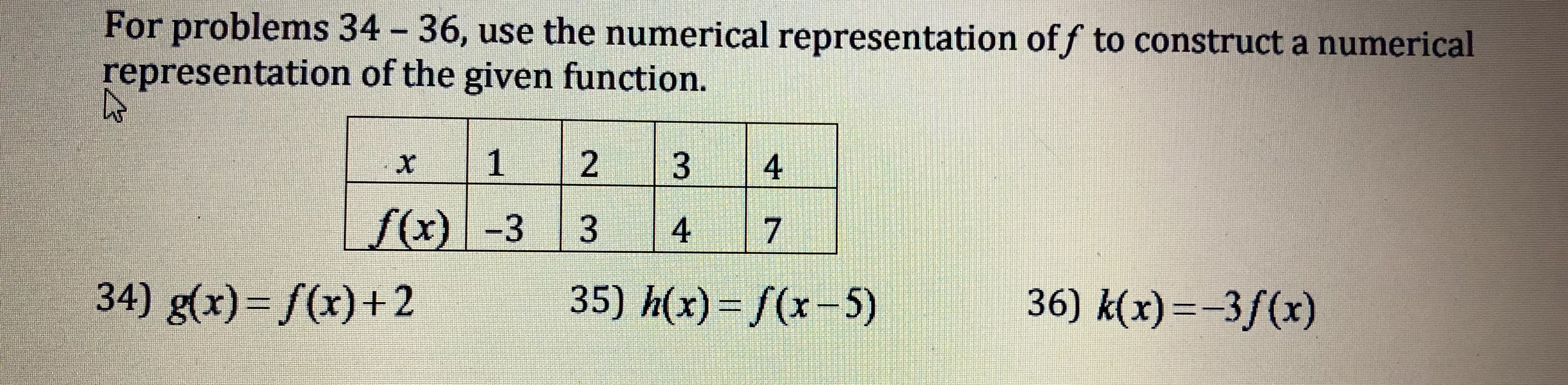For problems 34 -36, use the numerical representation of f to construct a numericalrepresentation of the given function.34) g(x) /(x)+235) h(x)-/(x -5)36) k(x)--3f(x)

Question

For 36help_outlineImage TranscriptioncloseFor problems 34 -36, use the numerical representation of f to construct a numerical representation of the given function. 34) g(x) /(x)+2 35) h(x)-/(x -5) 36) k(x)--3f(x) fullscreen
Step 1

36) Construct a numerical representation of the function k(x) = −3f(x) by using the numerical representation of f.

Step 2

Substitute x = 2 in k(x),

Step 3

Substitute x = 3 in k(x)...

Want to see the full answer?

See Solution

Want to see this answer and more?

Our solutions are written by experts, many with advanced degrees, and available 24/7

See Solution
Tagged in

Equations and In-equations